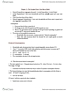# EC140 Chapter Notes - Chapter 27: Tax Rate, Autonomous Consumption, Consumption Function

54 views4 pages
School
Department
Coursebeigefly27 and 36717 others unlocked21
Verified Note
21 documents

## Document Summary

Ae = c + g + i + g + x m. The sum of the planned amounts of consumption expenditure (c), investment (i), government expenditure (g), and exports (x) minus the planned amount of imports (m) Disposable income equals real gdp minus net taxes (y-t). So if we replace yd with (y-t) the consumption function becomes: Using that equation to replace t in the consumption function, the consumption function becomes: Use the consumption function and the import function to replace c and m in the ae equation. Ae = (a-bta + i + g + x) + [b(1-t) m]y. Ae = a bta + b(1-t)y + i + g + x my. Occurs when aggregate planned expenditure (ae) equals real gdp (y) To calculate: solve the equations for the ae curve and the 45 line for the two unknown quantities ae and y. Replace ae with y in the ae equation to obtain. 1 [b (1-t) m] x a.